# Electromagnetism

In 1820, the Danish physicist, Hans Christian Oersted, discovered that the needle of a compass brought near a current carrying conductor would be deflected. When the current flow stopped, the compass needle returned to its original position. This important discovery demonstrated a relationship between electricity and magnetism that led to the electromagnet and to many of the inventions on which modern industry is based.

Oersted discovered that the magnetic field had no connection with the conductor in which the electrons were flowing, because the conductor was made of nonmagnetic copper. The electrons moving through the wire created the magnetic field around the conductor. Since a magnetic field accompanies a charged particle, the greater the current flow, the greater the magnetic field. Figure 1 illustrates the magnetic field around a current carrying wire.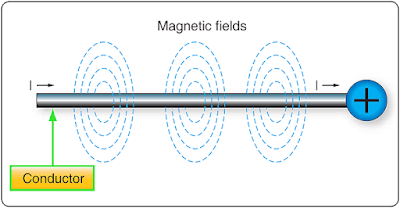Figure 1. Magnetic field formed around a conductor in which current is flowing

A series of concentric circles around the conductor represent the field, which if all the lines were shown would appear more as a continuous cylinder of such circles around the conductor.

As long as current flows in the conductor, the lines of force remain around it. [Figure 2] If a small current flows through the conductor, there will be a line of force extending out to circle A. If the current flow is increased, the line of force increases in size to circle B, and a further increase in current expands it to circle C. As the original line (circle) of force expands from circle A to B, a new line of force appears at circle A. As the current flow increases, the number of circles of force increases, expanding the outer circles farther from the surface of the current carrying conductor.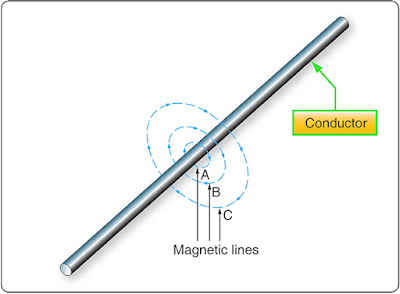Figure 2. Expansion of magnetic field as current increases

If the current flow is a steady nonvarying direct current, the magnetic field remains stationary. When the current stops, the magnetic field collapses and the magnetism around the conductor disappears.

A compass needle is used to demonstrate the direction of the magnetic field around a current carrying conductor. Figure 3A shows a compass needle positioned at right angles to, and approximately one inch from, a current carrying conductor. If no current were flowing, the north seeking end of the compass needle would point toward the earth’s magnetic pole. When current flows, the needle lines itself up at right angles to a radius drawn from the conductor. Since the compass needle is a small magnet, with lines of force extending from south to north inside the metal, it turns until the direction of these lines agrees with the direction of the lines of force around the conductor. As the compass needle is moved around the conductor, it maintains itself in a position at right angles to the conductor, indicating that the magnetic field around a current carrying conductor is circular. As shown in Figure 3B, when the direction of current flow through the conductor is reversed, the compass needle points in the opposite direction, indicating the magnetic field has reversed its direction.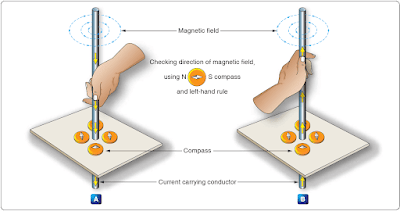Figure 3. Magnetic field around a current-carrying conductor

A method used to determine the direction of the lines of force when the direction of the current flow is known is shown in Figure 4. If the conductor is grasped in the left hand, with the thumb pointing in the direction of current flow, the fingers will be wrapped around the conductor in the same direction as the lines of the magnetic field. This is called the left-hand rule.Figure 4. Left-hand rule

Although it has been stated that the lines of force have direction, this should not be construed to mean that the lines have motion in a circular direction around the conductor. Although the lines of force tend to act in a clockwise or counterclockwise direction, they are not revolving around the conductor.

Since current flows from negative to positive, many illustrations indicate current direction with a dot symbol on the end of the conductor when the electrons are flowing toward and a plus sign when the current is flowing away from the observer. [Figure 5]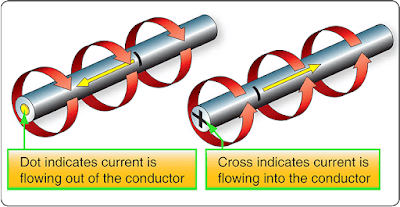Figure 5. Direction of current flow in a conductor

When a wire is bent into a loop and an electric current flows through it, the left-hand rule remains valid. [Figure 6] If the wire is coiled into two loops, many of the lines of force become large enough to include both loops. Lines of force go through the loops in the same direction, circle around the outside of the two coils, and come in at the opposite end. [Figure 7]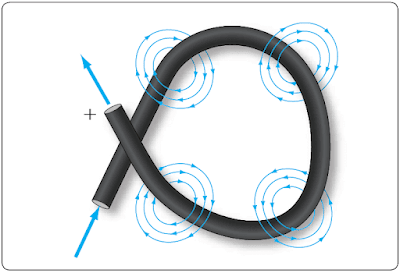Figure 6. Magnetic field around a looped conductor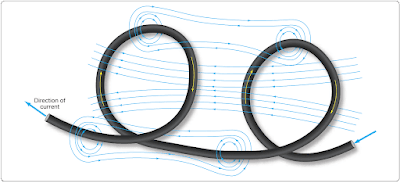Figure 7. Magnetic field around a conductor with two loops

When a wire contains many such loops, it is called a coil. The lines of force form a pattern through all the loops causing a high concentration of flux lines through the center of the coil. [Figure 8]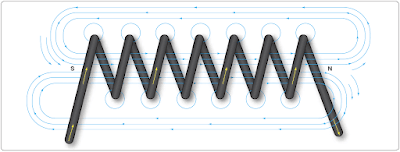Figure 8. Magnetic field of a coil

In a coil made from loops of a conductor, many of the lines of force are dissipated between the loops of the coil. By placing a soft iron bar inside the coil, the lines of force are concentrated in the center of the coil, since soft iron has a greater permeability than air. [Figure 9] This combination of an iron core in a coil of wire loops, or turns, is called an electromagnet, since the poles (ends) of the coil possess the characteristics of a bar magnet.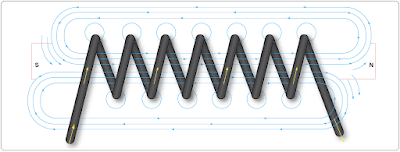Figure 9. Electromagnet

The addition of the soft iron core does two things for the current carrying coil. First, the magnetic flux is increased. Second, the flux lines are more highly concentrated.

When direct current flows through the coil, the core becomes magnetized with the same polarity (location of north and south poles) as the coil would have without the core. If the current is reversed, the polarity is also reversed.

The polarity of the electromagnet is determined by the left-hand rule in the same manner as the polarity of the coil without the core was determined. If the coil is grasped in the left hand in such a manner that the fingers curve around the coil in the direction of electron flow (minus to plus), the thumb points in the direction of the north pole. [Figure 10]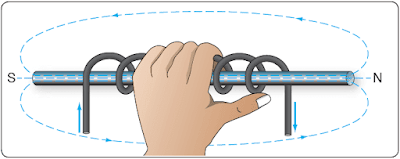Figure 10. Left-hand rule applied to a coil

The strength of the magnetic field of the electromagnet can be increased by either increasing the flow of current or the number of loops in the wire. Doubling the current flow approximately doubles the strength of the field. In a similar manner, doubling the number of loops approximately doubles magnetic field strength. Finally, the type of metal in the core is a factor in the field strength of the electromagnet.

A soft iron bar is attracted to either pole of a permanent magnet and, likewise, is attracted by a current carrying coil. The lines of force extend through the soft iron, magnetizing it by induction and pulling the iron bar toward the coil. If the bar is free to move, it is drawn into the coil to a position near the center where the field is strongest. [Figure 11]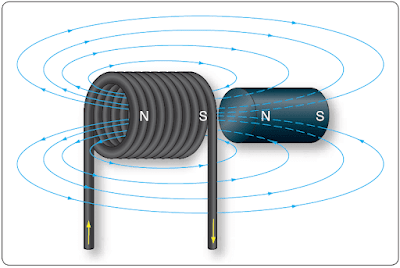Figure 11. Solenoid with iron core

Electromagnets are used in electrical instruments, motors, generators, relays, and other devices. Some electromagnetic devices operate on the principle that an iron core held away from the center of a coil is rapidly pulled into a center position when the coil is energized. This principle is used in the solenoid, also called solenoid switch or relay, in which the iron core is spring-loaded off center and moves to complete a circuit when the coil is energized.

RELATED POSTS